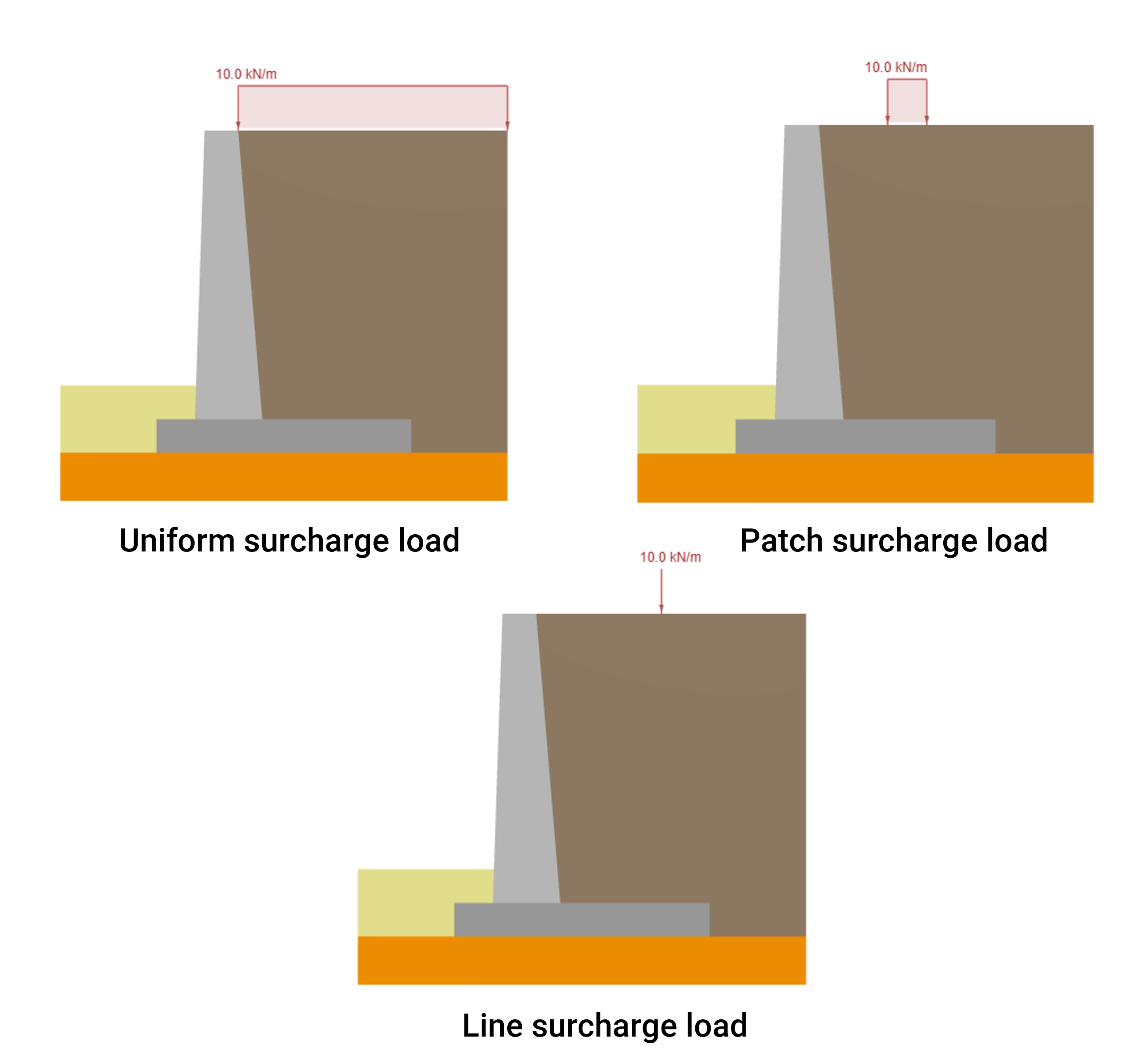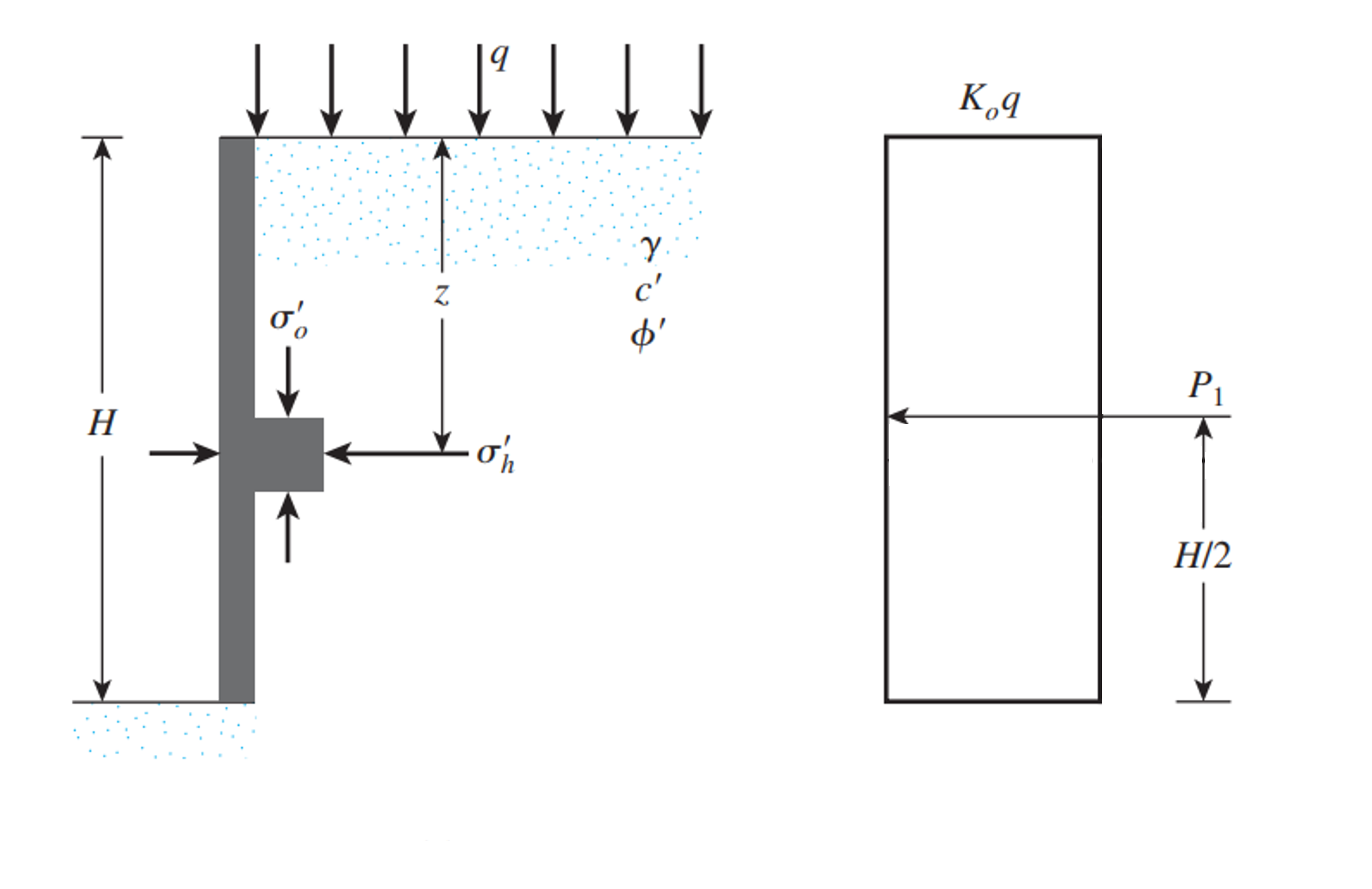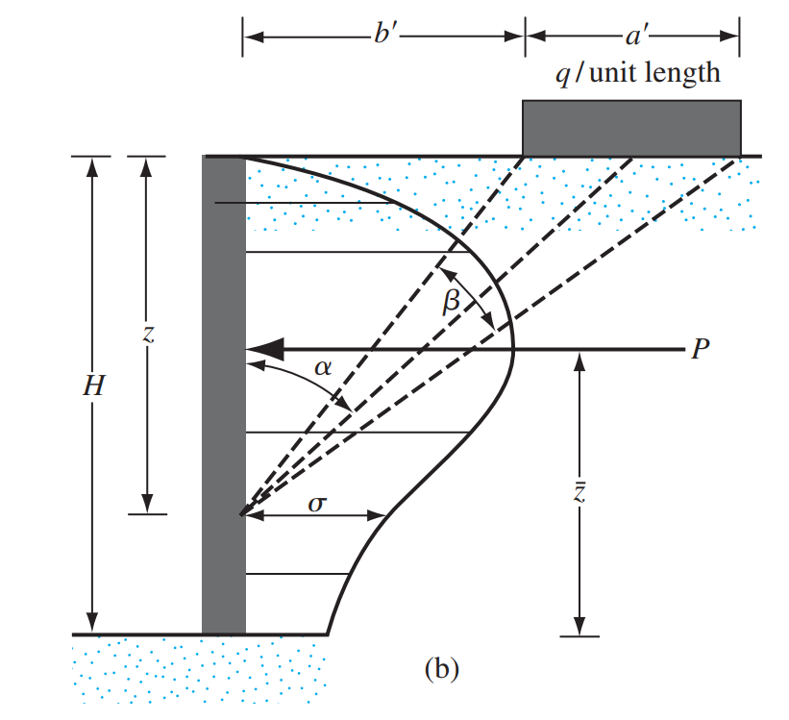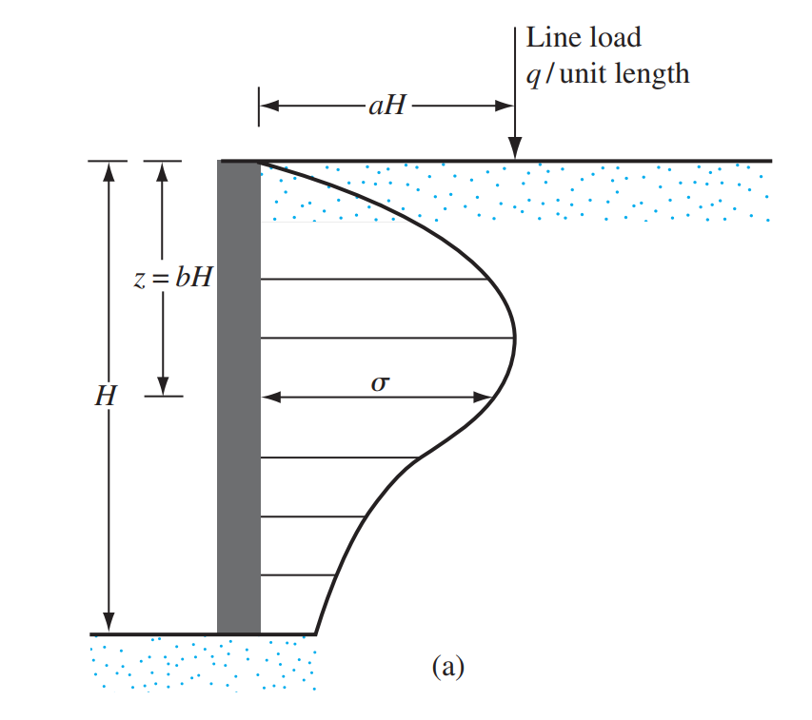SkyCivドキュメント

SkyCivソフトウェアのガイド - チュートリアル, ハウツーガイドと技術記事

1. ホーム
2. SkyCiv擁壁
3. 記事とチュートリアル
4. 過給荷重による側方土圧

# 過給荷重による側方土圧

## Calculating Lateral Earth Pressure due to Surcharge Loads on Retaining Wall

• 追加荷重による側方土圧の計算 3種類の重ね荷重により擁壁の背面に作用する側方土圧の計算方法に焦点を当てます。
• 3種類の重ね荷重により擁壁の背面に作用する側方土圧の計算方法に焦点を当てます。 3種類の重ね荷重により擁壁の背面に作用する側方土圧の計算方法に焦点を当てます。
• 3種類の重ね荷重により擁壁の背面に作用する側方土圧の計算方法に焦点を当てます。 3種類の重ね荷重により擁壁の背面に作用する側方土圧の計算方法に焦点を当てます。### 追加荷重による側方土圧の計算擁壁の両側の土壌ゾーンには、通常、いくつかの追加の外部荷重が適用されます

When the applied permanent or live surcharge load on the retaining wall is uniform and can be assumed that it is an infinite distributed load, 追加荷重による側方土圧の計算擁壁の両側の土壌ゾーンには、通常、いくつかの追加の外部荷重が適用されます. For that reason, the location of the resultant is in the middle of the wall’s total height. In the case of a sloped backfill, the pressure distribution skews an angle equal to the backfill inclination. この場合, the calculation of the resultant goes as follows:

• For soil in at-rest condition:

$$P_{dist, \; at-rest} = K_{0} \cdot q \cdot H_{土}$$

• cdot q cdot H_soil :

$$P_{dist, \; 下部構造土} = K_{a} \cdot q \cdot H_{土}$$

• cdot q cdot H_soil :

$$P_{dist, \; passive} = K_{p} \cdot q \cdot H_{土}$$Adapted from: それか, B. M. (2010). 基礎工学の原則, SI Edition. 章 7 Lateral Earth Pressure. Cengage Learning. 図 7.3

For the case of a patch or strip surcharge load on a retaining wall, the total force per unit length may be expressed as:

$$P_{パッチ} = frac{q}{90} [H(\theta_2-\theta_1)]$$

どこ,

$$\theta_1 = tan^{-1}(\フラク{b’}{H}) (君は)$$

$$\theta_2 = tan^{-1}(\フラク{a’ + b’}{H}) (君は)$$

This resultant force from the lateral earth pressure due to a patch or strip load is located at $$\バー{と}$$ measured from the bottom of the pressure distribution, $$\バー{と}$$ may be estimated using the following expression:

$$\バー{と} = H – [\フラク{H^2(\theta_2-\theta_1) + (R-Q) – 57.3a’H}{2H(\theta_2-\theta_1)}]$$

どこ $$R = (a’+b’)^2\cdot(90 – \theta_2)$$ そして $$Q = b’^2(90-\theta_1)$$Taken from: それか, B. M. (2010). 基礎工学の原則, SI Edition. 章 7 Lateral Earth Pressure. Cengage Learning. 図 7.14

$$\sigma = \frac{4q}{\pi H} \フラク{a^2 b}{(a^2 + b^2)^ 2}$$ ために $$a > 0.4$$

$$\sigma = \frac{q}{H} \フラク{0.203 b}{(0.16+ b^2)^ 2}$$ ために $$a \leq 0.4$$Taken from: それか, B. M. (2010). 基礎工学の原則, SI Edition. 章 7 Lateral Earth Pressure. Cengage Learning. 図 7.14

Integrating the expressions above from $$b = 0$$ に $$b = 1$$, it is possible to get the value of the resultant force due to the stress distributions of the applied line load:

$$P_{line} = \int_{0}^{H}\sigma \,dz = – \フラク{4 H^2 a^{2} q}{2 H^2 a^{2} \パイ + 2 H^2 \pi} + \フラク{2 q}{\パイ} = frac{2 q}{\pi \left(a^{2} + 1\正しい)}$$ ために $$a > 0.4$$

$$P_{line} = \int_{0}^{H}\sigma \,dz = 0.546875 q$$ ために $$a \leq 0.4$$

For calculating the location of the resultant force, the initial expressions for the stress at any depth are integrated over two intervals, one from $$b = 0$$ に $$b = b_r$$ and the other from $$b = b_r$$ に $$b = 1$$, after that, both expressions are plugged into an equation and its solution is the location of the resultant force from the lateral pressure distribution:

$$\バー{と} = H \left(1 – a \sqrt{\フラク{1}{2 a^{2} + 1}}\正しい)$$ ために $$a > 0.4$$

$$\バー{と} = H (1- 0.348155311911396)$$ ために $$a \leq 0.4$$

## 結論

Correctly estimating the lateral earth pressure resultant force due to superimposed loads and its location is a crucial step in the Retaining Wall Design Process. For more information about how this lateral earth pressure is included in the Retaining Wall Design Process, refer to the article ここに.

Apart from the lateral earth pressure due to surcharge loads, the self-weight of the soil also exerts pressure on the Retaining Wall’s backface, the details of how to calculate this pressure for different soil conditions like at-rest, 下部構造土, and passive are discussed in another article ここに.

## 参考文献

それか, B. M. (2010). 基礎工学の原則, SI Edition. 章 7 Lateral Earth Pressure. Cengage Learning.

## 擁壁計算機

SkyCivは、壁の側方土圧を計算する無料の擁壁計算機を提供します, 擁壁の安定性分析を実行します. の場合、ベースの下部から壁の高さの半分, の場合、ベースの下部から壁の高さの半分, 転倒に対する擁壁の安定性を計算する方法, スライディング, とベアリング!オスカーサンチェス

ベン (民事)